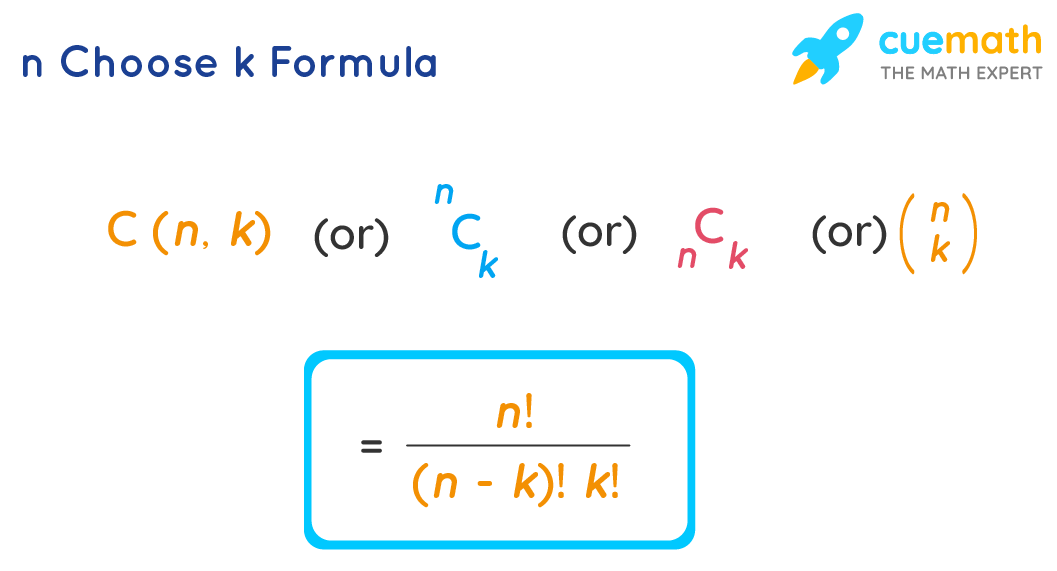# n Choose k Formula

"Choose" means "select". "n choose k formula" is used to find the number of ways selecting k things out of n things. Sometimes we prefer selecting but we do not care about ordering them. For example, you are packing luggage for a trip, and you want to carry 5 dresses out of 10 new dresses you have. This can be done in various ways, it doesn't matter in which order you have chosen them but it all matters what dresses you have chosen. Let us learn the n choose k formula along with a few solved examples.

## What is n Choose k Formula?

"n choose k" is denoted by C (n, k) (or) $$n_{ C_{k}}$$ (or) $$_{n} C_{k}$$ (or) $$\left(\begin{array}{l}n \\k\end{array}\right)$$. It is also known as a binomial coefficient. It is used to find the number of ways of selecting k different things from n different things. The n choose k formula is also known as combinations formula (as we call a way of choosing things to be a combination). This formula involves factorials.

The n Choose k Formula is:

C (n , k) = n! / [ (n-k)! k! ]## Solved Examples Using n Choose k Formula

### Find the number of ways of forming a team of 5 members out of 10 members.

Solution

To find: The number of ways of forming a team of 5 members out of 10 members.

Here, the total number of members is n = 10.

The number of members to be selected to form a team is k = 5.

This can be done in C(n , k) ways and we will find this using the n choose k formula.

C (n , k) = n! / [ (n-k)! k! ]

C (10, 5) = 10! / [ (10 - 5)! 5! ]

C(10, 5) = (10 × 9 × 8 × 7 × 6 × 5!) / (5! 5!)

C(10, 5) = (10 × 9 × 8 × 7 × 6) / (5 × 4 × 3 × 2 × 1)

C(10, 5) = 252.

Answer: The number of ways of forming a team of 5 members = 252.

### There are 50 people at a party. Among them, each person shakes hand with each of the others exactly once. Then how many handshakes are supposed to happen there?

Solution

To find: The total number of shake hands at the party.

The total number of people at the party is n = 50.

For a shaking hand to happen, there must be two people.

So the number of shake hands is equal to the number of ways of selecting 2 people out of 50 people. So k = 2.

Using the n choose k formula,

C (n , k) = n! / [ (n-k)! k! ]

C (50, 2) = 50! / [ (50 - 2)! 2! ]

C (50, 2) = (50 × 49 × 48!) / (48! 2!)

C (50, 2) = (50 × 49) / (2 × 1)

C (50, 2) = 1225.

Answer: The number of shake hands = 1,225.

Learn from the best math teachers and top your exams

• Live one on one classroom and doubt clearing
• Practice worksheets in and after class for conceptual clarity
• Personalized curriculum to keep up with school